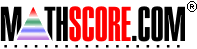Math Practice Online > free > lessons > Florida > 6th grade > Parallelogram Area

## Parallelogram Area

Calculate the area of a parallelogram and express it in the correct units.

 Sample Problems for Parallelogram Area Lesson for Parallelogram Area

### This topic aligns to the following state standards

Grade 4: Geo 2. Applies the concepts of area and perimeter to solve real-world and mathematical problems.
Grade 7: Meas 3. Solves and explains problems involving perimeter, area, and circumference.

Copyright Accurate Learning Systems Corporation 2008.